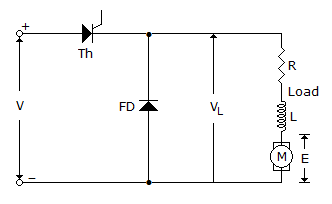# Electronics and Communication Engineering - Power Electronics

Exercise : Power Electronics - Section 4
16.
In a 3 phase bridge inverter with 180° mode of operation the numbers of thyristors conducting at one time are
1
2
3
4
Explanation:
No answer description is available. Let's discuss.

17.
A single phase full converter can operate in
Explanation:
No answer description is available. Let's discuss.

18.
In a 3 phase semiconverter, the freewheeling diode comes into operation only if firing angle
is zero
is 60°
is more than 60°
is more than 90°
Explanation:
No answer description is available. Let's discuss.

19.
In the circuit of figure, the load current when thyristor is offis constant
increases exponentially
decreases exponentially
zero